## Yan Han's blog

On Computer Technology

 Tags

# Analysis of the Adult data set from UCI Machine Learning Repository¶

This is an analysis of the Adult data set in the UCI Machine Learning Repository.

This data set is meant for binary class classification - to predict whether the income of a person exceeds 50K per year based on some census data.

Our objective here is to familiarize ourselves with scikit-learn by training a Logistic Regression classifier on the data set by using grid search, cross validation and pipeline, and see how well we can do.

At the end of it all, I decided to do a writeup using a Jupyter notebook (which I have pretty much no experience with), so here we are. For those of you reading the HTML version of this notebook, you might have to scroll quite a bit because I have no idea how to toggle the scrolling for the HTML export.

Gist with ipynb file and data set: https://gist.github.com/yanhan/355fb068eb5089b4de78b8de326e6358

## Preliminaries¶

First, we import the necessary libraries:

In :
from IPython.display import display
from numpy.random import RandomState
from sklearn.linear_model import LogisticRegression, LogisticRegressionCV
from sklearn.metrics import accuracy_score, confusion_matrix, make_scorer, precision_recall_fscore_support, roc_auc_score
from sklearn.model_selection import GridSearchCV, StratifiedKFold, train_test_split
from sklearn.pipeline import Pipeline
from sklearn.preprocessing import RobustScaler, StandardScaler
from sklearn_pandas import DataFrameMapper

import numpy as np
import pandas as pd


We create a numpy.random.RandomState so that we can reproduce the same results each time we run this notebook.

In :
rs = RandomState(130917)


## Exploratory Data Analysis¶

This dataset is small and consists of 48842 rows with 14 columns (not counting the column giving the response variable). Hence we can load it entirely into memory. The fields of this data set are delimited by spaces; we can make use of pandas read_csv function to load it into memory as a dataframe.

In :
df = pd.read_csv("Dataset.data", header=None, delimiter=r"\s+",)


Let's take a look at the first few rows of the dataframe.

In :
print(df.head())

   0          1       2             3   4                   5   \
0  25    Private  226802          11th   7       Never-married
1  38    Private   89814       HS-grad   9  Married-civ-spouse
2  28  Local-gov  336951    Assoc-acdm  12  Married-civ-spouse
3  44    Private  160323  Some-college  10  Married-civ-spouse
4  18          ?  103497  Some-college  10       Never-married

6          7      8       9     10  11  12             13  \
0  Machine-op-inspct  Own-child  Black    Male     0   0  40  United-States
1    Farming-fishing    Husband  White    Male     0   0  50  United-States
2    Protective-serv    Husband  White    Male     0   0  40  United-States
3  Machine-op-inspct    Husband  Black    Male  7688   0  40  United-States
4                  ?  Own-child  White  Female     0   0  30  United-States

14
0  <=50K
1  <=50K
2   >50K
3   >50K
4  <=50K


Seems like everything is as according to the specifications. For ease of human consumption, we assign column names to the dataframe based on the specs.

In :
df.columns = [
"Age", "WorkClass", "fnlwgt", "Education", "EducationNum",
"MaritalStatus", "Occupation", "Relationship", "Race", "Gender",
"CapitalGain", "CapitalLoss", "HoursPerWeek", "NativeCountry", "Income"
]


See if there are any NaNs in the dataframe:

In :
df.isnull().values.any()

Out:
False

Let's show the first few rows of the dataframe with the column names:

In :
print(df.head())

   Age  WorkClass  fnlwgt     Education  EducationNum       MaritalStatus  \
0   25    Private  226802          11th             7       Never-married
1   38    Private   89814       HS-grad             9  Married-civ-spouse
2   28  Local-gov  336951    Assoc-acdm            12  Married-civ-spouse
3   44    Private  160323  Some-college            10  Married-civ-spouse
4   18          ?  103497  Some-college            10       Never-married

Occupation Relationship   Race  Gender  CapitalGain  CapitalLoss  \
0  Machine-op-inspct    Own-child  Black    Male            0            0
1    Farming-fishing      Husband  White    Male            0            0
2    Protective-serv      Husband  White    Male            0            0
3  Machine-op-inspct      Husband  Black    Male         7688            0
4                  ?    Own-child  White  Female            0            0

HoursPerWeek  NativeCountry Income
0            40  United-States  <=50K
1            50  United-States  <=50K
2            40  United-States   >50K
3            40  United-States   >50K
4            30  United-States  <=50K


Let's take a look at the values of the Income column:

In :
df.Income.unique()

Out:
array(['<=50K', '>50K'], dtype=object)

All good. Let's convert the <=50Ks into -1 and the >50K into +1

In :
df["Income"] = df["Income"].map({ "<=50K": -1, ">50K": 1 })


Let's extract the response variable into a numpy array and drop it from the dataframe:

In :
y_all = df["Income"].values
df.drop("Income", axis=1, inplace=True,)


Let's look at the data again:

In :
print(df.head())

   Age  WorkClass  fnlwgt     Education  EducationNum       MaritalStatus  \
0   25    Private  226802          11th             7       Never-married
1   38    Private   89814       HS-grad             9  Married-civ-spouse
2   28  Local-gov  336951    Assoc-acdm            12  Married-civ-spouse
3   44    Private  160323  Some-college            10  Married-civ-spouse
4   18          ?  103497  Some-college            10       Never-married

Occupation Relationship   Race  Gender  CapitalGain  CapitalLoss  \
0  Machine-op-inspct    Own-child  Black    Male            0            0
1    Farming-fishing      Husband  White    Male            0            0
2    Protective-serv      Husband  White    Male            0            0
3  Machine-op-inspct      Husband  Black    Male         7688            0
4                  ?    Own-child  White  Female            0            0

HoursPerWeek  NativeCountry
0            40  United-States
1            50  United-States
2            40  United-States
3            40  United-States
4            30  United-States


Now, the Age, fnlwgt, EducationNum, CapitalGain, CapitalLoss and HoursPerWeek are clearly numerical. Let's get some summary statistics on these numerical columns:

In :
df.describe()

Out:
Age fnlwgt EducationNum CapitalGain CapitalLoss HoursPerWeek
count 48842.000000 4.884200e+04 48842.000000 48842.000000 48842.000000 48842.000000
mean 38.643585 1.896641e+05 10.078089 1079.067626 87.502314 40.422382
std 13.710510 1.056040e+05 2.570973 7452.019058 403.004552 12.391444
min 17.000000 1.228500e+04 1.000000 0.000000 0.000000 1.000000
25% 28.000000 1.175505e+05 9.000000 0.000000 0.000000 40.000000
50% 37.000000 1.781445e+05 10.000000 0.000000 0.000000 40.000000
75% 48.000000 2.376420e+05 12.000000 0.000000 0.000000 45.000000
max 90.000000 1.490400e+06 16.000000 99999.000000 4356.000000 99.000000

Age, fnlwgt, EducationNum and HoursPerWeek look pretty ok. But CapitalGain and CapitalLoss both have an IQR of 0. Could they be power law distributions? Let's find out.

In :
df.CapitalGain.value_counts()

Out:
0        44807
15024      513
7688       410
7298       364
99999      244
3103       152
5178       146
5013       117
4386       108
8614        82
3325        81
2174        74
10520       64
4650        63
27828       58
4064        54
594         52
3137        51
20051       49
14084       49
3908        42
6849        42
13550       42
2829        42
1055        37
4787        35
3411        34
14344       34
3464        33
2597        31
...
9562         5
2538         5
6723         5
2050         5
11678        4
2936         4
7896         4
4931         4
4687         4
2961         4
1455         4
1424         4
3432         4
2062         3
2993         3
2009         3
6360         3
41310        3
6097         2
5060         2
18481        2
1264         2
7978         2
7262         1
1731         1
2387         1
22040        1
6612         1
1111         1
1639         1
Name: CapitalGain, dtype: int64

Alright, a whopping 91.7% of the CapitalGain consists of 0. Now onto the CapitalLoss column:

In :
df.CapitalLoss.value_counts()

Out:
0       46560
1902      304
1977      253
1887      233
2415       72
1485       71
1848       67
1590       62
1602       62
1876       59
1740       58
1672       50
1741       44
1564       43
2258       39
1719       38
1980       36
2001       35
1408       35
1669       35
2002       33
1579       30
2051       29
1974       28
1721       28
2339       27
1504       26
2377       25
1628       24
1762       20
...
1411        4
4356        3
419         3
1944        3
2267        3
1510        3
1735        3
1429        3
1844        3
1648        3
3175        2
2163        2
2467        2
3683        2
2352        2
2754        2
2282        2
3900        2
810         2
1755        2
974         2
2080        1
2465        1
1911        1
155         1
1539        1
2489        1
2201        1
1421        1
1870        1
Name: CapitalLoss, dtype: int64

This is even worse. 95.3% of the CapitalLoss column consists of 0.

Frankly, I do not know how to handle columns distributed according to power law, so I choose to drop them:

In :
df.drop("CapitalGain", axis=1, inplace=True,)
df.drop("CapitalLoss", axis=1, inplace=True,)


Let's convert the Age, fnlwgt, EducationNum and HoursPerWeek to floating point so scikit-learn / numpy won't complain about scaling later.

In :
df.Age = df.Age.astype(float)
df.fnlwgt = df.fnlwgt.astype(float)
df.EducationNum = df.EducationNum.astype(float)
df.HoursPerWeek = df.HoursPerWeek.astype(float)


Time for the categorical variables. First up WorkClass:

In :
df.WorkClass.unique()

Out:
array(['Private', 'Local-gov', '?', 'Self-emp-not-inc', 'Federal-gov',
'State-gov', 'Self-emp-inc', 'Without-pay', 'Never-worked'], dtype=object)

Next up Education:

In :
df.Education.unique()

Out:
array(['11th', 'HS-grad', 'Assoc-acdm', 'Some-college', '10th',
'Prof-school', '7th-8th', 'Bachelors', 'Masters', 'Doctorate',
'5th-6th', 'Assoc-voc', '9th', '12th', '1st-4th', 'Preschool'], dtype=object)

Next up MaritalStatus:

In :
df.MaritalStatus.unique()

Out:
array(['Never-married', 'Married-civ-spouse', 'Widowed', 'Divorced',
'Separated', 'Married-spouse-absent', 'Married-AF-spouse'], dtype=object)

Next up Occupation:

In :
df.Occupation.unique()

Out:
array(['Machine-op-inspct', 'Farming-fishing', 'Protective-serv', '?',
'Exec-managerial', 'Tech-support', 'Sales', 'Priv-house-serv',
'Transport-moving', 'Handlers-cleaners', 'Armed-Forces'], dtype=object)

Next up Relationship:

In :
df.Relationship.unique()

Out:
array(['Own-child', 'Husband', 'Not-in-family', 'Unmarried', 'Wife',
'Other-relative'], dtype=object)

Next up Race:

In :
df.Race.unique()

Out:
array(['Black', 'White', 'Asian-Pac-Islander', 'Other',
'Amer-Indian-Eskimo'], dtype=object)

Next up Gender:

In :
df.Gender.unique()

Out:
array(['Male', 'Female'], dtype=object)

Next up NativeCountry:

In :
df.NativeCountry.unique()

Out:
array(['United-States', '?', 'Peru', 'Guatemala', 'Mexico',
'Dominican-Republic', 'Ireland', 'Germany', 'Philippines',
'South', 'Columbia', 'Japan', 'India', 'Cambodia', 'Poland', 'Laos',
'England', 'Cuba', 'Taiwan', 'Italy', 'Canada', 'Portugal', 'China',
'Nicaragua', 'Honduras', 'Iran', 'Scotland', 'Jamaica', 'Ecuador',
'Outlying-US(Guam-USVI-etc)', 'France', 'Holand-Netherlands'], dtype=object)

Looks like there's more unique values for NativeCountry compared to the other categorical variables. Exactly how many unique values are there?

In :
len(df.NativeCountry.unique())

Out:
42

It is not entirely clear what Relationship means in this data set, since there is already a MaritalStatus column. While it makes sense for someone whose MaritalStatus column has the value Married-civ-spouse or Married-AF-spouse (whatever those 2 values mean) to have Relationship column with value Husband or Wife, can someone's MaritalStatus column have the value Married-civ-spouse but whose Relationship column have value Own-child (whatever that means)?

Common sense tells us that WorkClass, Education, Occupation are features with good predictive power with regards to one's income. Similarly for Race and Gender but to a slightly lesser extent. But how about MaritalStatus and the not so clear Relationship column?

What about NativeCountry? A possible argument for this column is that there may be a higher concentration of people from certain countries in certain occupations. And we know that occupations certainly influence income. But doesn't that make NativeCountry redundant? Not entirely. If it is possible for there to be a non-trivial difference between the average income of men and women (with all else equal), then it is possible for there to be a difference between the average income of people from the US and people not from the US who are in the same occupation (again all else being equal). Hence this feature could have predictive power as well.

Long story short, we choose to retain all these columns and use one-hot encoding to transform them into numerical features that the Logistic Regression model we're using later can consume.

In :
df = pd.get_dummies(df, columns=[
"WorkClass", "Education", "MaritalStatus", "Occupation", "Relationship",
"Race", "Gender", "NativeCountry",
])


What are the dimensions of our dataframe?

In :
df.shape

Out:
(48842, 106)

## Splitting the data for training and testing¶

Is our data imbalanced towards one class?

In :
pd.value_counts(pd.Series(y_all))

Out:
-1    37155
1    11687
dtype: int64

Turns out, yes. Only 23.9% of the data belongs to the positive class. This is something we'll have to keep in mind when training the classifier later.

We'll do a stratified split of the data set into a training set and a test set, with 25% of the data going into the test set. A stratified split will ensure that the percentage of every class in the training set and test set is very similar to that of the entire data set.

Recall the RandomState variable in rs that we created right at the start of the file? We pass that in to the random_state argument of the train_test_split function:

In :
X_train, X_test, y_train, y_test = train_test_split(
df, y_all, test_size=0.25, stratify=y_all, random_state=rs,
)


## The Machine Learning part¶

Recall that we have 4 numerical columns: Age, fnlwgt, EducationNum and HoursPerWeek. We will have to scale the data. For simplicity, we choose to use StandardScaler. No scaling / transformation will be done for the rest of the columns. We use the DataFrameMapper from the sklearn-pandas package to accomplish this.

In :
standard_scaler_cols = ["Age", "fnlwgt", "EducationNum", "HoursPerWeek",]
other_cols = list(set(df.columns) - set(standard_scaler_cols))
mapper = DataFrameMapper(
[([col,], StandardScaler(),) for col in standard_scaler_cols] +
[(col, None,) for col in other_cols]
)


Now we create our pipeline, which will consist of 2 steps:

1. Scaling the 4 numerical columns using DataFrameMapper
2. Training a Logistic Regression classifier

The RandomState variable rs is passed into the Logistic Regression classifier.

In :
clf = LogisticRegression(random_state=rs,)
pipeline = Pipeline([
("scale", mapper,),
("logit", clf,)
])


We are going to perform a grid search with cross validation over 2 "hyperparameters":

1. The C argument to LogisticRegression indicating the inverse of the regularization strength. We're going to vary this from 1e-04 to 1e4 with a 10x increase from one value to the next. This is a legit hyperparameter.
2. The class_weight argument to LogisticRegression. Seems like setting this to balanced could help out in our case where the classes are imbalanced. Not sure whether this is a real hyperparameter to Logistic Regression which is why I put the hyperparameters in quotes.

For scoring, we will make use of roc_auc_score instead of the default accuracy, because of the imbalance in classes. ROC AUC score also has an intuitive appeal in that it represents the probability of the classifier ranking a randomly selected positive instance over a randomly selected negative instance

To make the results repeatable, we create a StratifiedKFold object and pass it the RandomState variable rs. Then we pass the StratifiedKFold object as the cross validation generator via the cv argument of the GridSearchCV object.

In :
strat_kfold = StratifiedKFold(10, random_state=rs,)
estimator = GridSearchCV(
pipeline,
param_grid={
"logit__C": np.power(10, np.arange(-4.0, 5.0)),
"logit__class_weight": ["balanced", None,],
},
scoring=make_scorer(roc_auc_score),
cv=strat_kfold,
)


Now we perform the grid search with cross validation and print out the results.

I chose to disable this warning because there's so many of them:

DeprecationWarning: Estimator DataFrameMapper modifies parameters in __init__. This behavior is deprecated as of 0.18 and support for this behavior will be removed in 0.20.

It should have been resolved in one of the issues for the sklearn-pandas package but for some reason it has resurfaced.

Credits to this Stack Overflow answer

In :
import warnings
warnings.filterwarnings("ignore")
estimator.fit(X_train, y_train)

Out:
GridSearchCV(cv=StratifiedKFold(n_splits=10,
random_state=<mtrand.RandomState object at 0x7fda6e21e438>,
shuffle=False),
error_score='raise',
estimator=Pipeline(steps=[('scale', DataFrameMapper(default=False, df_out=False,
features=[(['Age'], StandardScaler(copy=True, with_mean=True, with_std=True)), (['fnlwgt'], StandardScaler(copy=True, with_mean=True, with_std=True)), (['EducationNum'], StandardScaler(copy=True, with_mean=True, with_std=True)), (... object at 0x7fda6e21e438>,
solver='liblinear', tol=0.0001, verbose=0, warm_start=False))]),
fit_params={}, iid=True, n_jobs=1,
param_grid={'logit__C': array([  1.00000e-04,   1.00000e-03,   1.00000e-02,   1.00000e-01,
1.00000e+00,   1.00000e+01,   1.00000e+02,   1.00000e+03,
1.00000e+04]), 'logit__class_weight': ['balanced', None]},
pre_dispatch='2*n_jobs', refit=True, return_train_score=True,
scoring=make_scorer(roc_auc_score), verbose=0)

Let's look at the results in a pandas DataFrame:

In :
cv_results_df = pd.DataFrame(estimator.cv_results_)

Out:
mean_fit_time mean_score_time mean_test_score mean_train_score param_logit__C param_logit__class_weight params rank_test_score split0_test_score split0_train_score ... split7_test_score split7_train_score split8_test_score split8_train_score split9_test_score split9_train_score std_fit_time std_score_time std_test_score std_train_score
4 0.166931 0.010015 0.806727 0.807109 0.01 balanced {'logit__C': 0.01, 'logit__class_weight': 'bal... 1 0.820290 0.805417 ... 0.807479 0.806635 0.802130 0.808239 0.808735 0.807573 0.002707 0.000833 0.009891 0.001270
6 0.194077 0.009795 0.806065 0.807942 0.1 balanced {'logit__C': 0.1, 'logit__class_weight': 'bala... 2 0.821777 0.806334 ... 0.805752 0.808329 0.804611 0.808550 0.804007 0.808412 0.001292 0.000393 0.010309 0.000976
8 0.258479 0.013917 0.805728 0.807752 1 balanced {'logit__C': 1.0, 'logit__class_weight': 'bala... 3 0.819529 0.805887 ... 0.805752 0.808108 0.803469 0.808626 0.803975 0.808495 0.025620 0.011498 0.010077 0.000990
10 0.349818 0.010560 0.804717 0.807950 10 balanced {'logit__C': 10.0, 'logit__class_weight': 'bal... 4 0.818389 0.806247 ... 0.805573 0.808421 0.801969 0.808776 0.804154 0.808249 0.043081 0.001983 0.009919 0.000946
14 0.413492 0.013878 0.804699 0.807990 1000 balanced {'logit__C': 1000.0, 'logit__class_weight': 'b... 5 0.818389 0.806267 ... 0.805752 0.808465 0.801969 0.808859 0.804154 0.808416 0.084363 0.011494 0.009904 0.000984

5 rows × 32 columns

Best parameters for Logistic Regression:

In :
print(estimator.best_params_)

{'logit__C': 0.01, 'logit__class_weight': 'balanced'}


Ok, so setting class_weight to balanced does help for Logistic Regression. The best regularization C is 0.01.

Now we write a function to build a pandas DataFrame from a confusion matrix:

In :
def _build_df_from_confusion_matrix(confusion_matrix, as_fractions=False):
if as_fractions:
x = np.array(confusion_matrix)
x = np.apply_along_axis(
lambda row: [
row / (row + row),
row / (row + row)
],
1,
x
)
else:
x = confusion_matrix
df = pd.DataFrame(
x,
index=["<= 50K", "> 50K"],
columns=["<= 50K", "> 50K"]
)
df.index.names = ["Actual"]
df.columns.names = ["Predicted"]
return df


### Performance on training set¶

In :
y_train_predicted = estimator.predict(X_train)
print("Training set accuracy score: {}".format(
accuracy_score(y_train, y_train_predicted)
))
print("Training set AUROC score: {}".format(estimator.score(X_train, y_train)))
print("\nConfusion matrix for training set:")
training_confusion_matrix = confusion_matrix(
y_train, y_train_predicted
)
display(_build_df_from_confusion_matrix(training_confusion_matrix))
print("Same as above but in fractions:")
display(_build_df_from_confusion_matrix(training_confusion_matrix, as_fractions=True))
print("Precision, recall, f-score:")
precision_recall_fscore_support(y_train, y_train_predicted)

Training set accuracy score: 0.785755234637329
Training set AUROC score: 0.8071775040759195

Confusion matrix for training set:

Predicted <= 50K > 50K
Actual
<= 50K 21348 6518
> 50K 1330 7435
Same as above but in fractions:

Predicted <= 50K > 50K
Actual
<= 50K 0.766095 0.233905
> 50K 0.151740 0.848260
Precision, recall, f-score:

Out:
(array([ 0.94135285,  0.53286032]),
array([ 0.76609488,  0.84826013]),
array([ 0.84472934,  0.65454706]),
array([27866,  8765]))

While the AUROC score of 0.8071775040759195 seems decent, the precision, recall and fscore tells a different story.

We see that the precision for the negative class is awesome (94.135%) but precision for the positive class sucks (53.286%). Even though 76.6% of the negative class instances were classified correctly, the sheer number of instances belonging to the negative class means that overall we get a very high false positive rate of 46.7%.

That said, the recall for the positive class is 84.826%. So this classifier captures a good part of the instances that belong to the positive class.

I have no idea how to interpret the f-score and I have no time to learn how to do it so I will skip that.

But this is for the training set. How about the test set?

### Performance on test set¶

In :
y_test_predicted = estimator.predict(X_test)
print("Test set accuracy score: {}".format(
accuracy_score(y_test, y_test_predicted)
))
print("Test set AUROC score: {}".format(estimator.score(X_test, y_test)))
print("\nConfusion matrix for test set:")
test_confusion_matrix = confusion_matrix(y_test, y_test_predicted)
display(_build_df_from_confusion_matrix(test_confusion_matrix))
print("Same as above but in fractions:")
display(_build_df_from_confusion_matrix(test_confusion_matrix, as_fractions=True))
print("Precision, recall, f-score:")
precision_recall_fscore_support(y_test, y_test_predicted)

Test set accuracy score: 0.7903529604455
Test set AUROC score: 0.8116512329133934

Confusion matrix for test set:

Predicted <= 50K > 50K
Actual
<= 50K 7160 2129
> 50K 431 2491
Same as above but in fractions:

Predicted <= 50K > 50K
Actual
<= 50K 0.770804 0.229196
> 50K 0.147502 0.852498
Precision, recall, f-score:

Out:
(array([ 0.94322224,  0.53917749]),
array([ 0.77080418,  0.85249829]),
array([ 0.84834123,  0.66056749]),
array([9289, 2922]))

We get very similar numbers for the test set. Seems like the classifier performed slightly better for the test set than the training set. Which is kind of strange. But I guess this means that the Logistic Regression algorithm has learnt something from the data.

So we have a classifier with good recall but bad precision. This is great if we want to identify as many people with income exceeding 50K per annum as possible, but it is not so great when we realize that almost half the people identified as income exceeding 50K per annum actually earn less than 50K per annum.

This not so great performance could be mainly attributed to us dropping the CapitalGain and CapitalLoss columns. I'm not entirely sure how to deal with those but an idea is to take logarithm of those columns - we can handle zeros by adding 1 to the entire column.

Another idea is to add polynomial features. This can be done without too much effort in scikit-learn. The major downside is that we already had 106 features after dropping the CapitalGain and CapitalLoss columns. With those 2 columsn we will have 108 features. With polynomial features, this number can easily triple. Grid search will take a lot more time to complete.

## Conclusion¶

We learnt how to:

1. train a Logistic Regression classifier using grid search with cross validation to select for hyperparameters and a pipeline that does scaling only on the validation set (for each fold)
2. make use of DataFrameMapper in the sklearn-pandas package
3. use the sklearn.metrics.roc_auc_score as the evaluation criteria during GridSearchCV
4. use some pandas
5. use Jupyter notebooks (so awesome)
6. better interpret the confusion matrix when used in conjunction with precision and recall. Accuracy is not everything if the classifier is not discriminative
7. deal with a slightly bigger data set than the last time

A pretty good deal I'd say. The next time, I will perform some analysis / learning on a 'more real' dataset.

## Addendum - Making use of the CapitalGain and CapitalLoss features¶

One fine day I recalled seeing that there are no negative values in the CapitalGain and CapitalLoss columns - the minimum value is zero. Perhaps we can do take logarithms and see how the resulting values are distributed.

In :
# Same code from above to re create the dataframe
df.columns = [
"Age", "WorkClass", "fnlwgt", "Education", "EducationNum",
"MaritalStatus", "Occupation", "Relationship", "Race", "Gender",
"CapitalGain", "CapitalLoss", "HoursPerWeek", "NativeCountry", "Income"
]
%matplotlib inline
import matplotlib.pyplot as plt

log_capital_gain = np.log(df.CapitalGain + 1)
print("Min: {}, Max: {}".format(
np.min(log_capital_gain), np.max(log_capital_gain)
))
hist, bins = np.histogram(log_capital_gain, bins=50)
plt.bar((bins[:-1] + bins[1:]) / 2, hist, align="center",)
plt.xlabel("log(CapitalGain)")
plt.ylabel("Counts")

Min: 0.0, Max: 11.512925464970229

Out:
<matplotlib.text.Text at 0x7fdad9321358>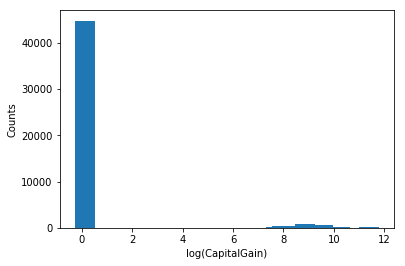In :
log_capital_loss = np.log(df.CapitalLoss + 1)
print("Min: {}, Max: {}".format(
np.min(log_capital_loss), np.max(log_capital_loss)
))
hist, bins = np.histogram(log_capital_loss, bins=50)
plt.bar((bins[:-1] + bins[1:]) / 2, hist, align="center",)
plt.xlabel("log(CapitalLoss)")
plt.ylabel("Counts")

Min: 0.0, Max: 8.379539026117442

Out:
<matplotlib.text.Text at 0x7fdad8e42c88>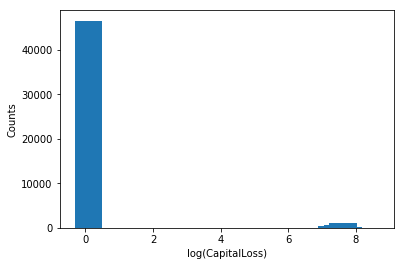Hmm so I remembered the wrong stuff. After scaling, the values in these 2 columns will still be distributed very similarly as before the scaling is done. A log-log plot of both the counts and the values should show approximately a linear trend. Let's do that.

In :
len_of_range = np.max(df.CapitalLoss) + 1
m = np.hstack(
(
np.array(range(len_of_range)).reshape((len_of_range, 1,)),
np.bincount(df.CapitalLoss).reshape((len_of_range, 1,)),
)
)
m = m[m[:, 1] > 0]
m[:, 0] += 1
m = np.log(m)
plt.plot(m[:, 0], m[:, 1])
plt.xlabel("log(CapitalLoss + 1)")
plt.ylabel("log(Counts)")

Out:
<matplotlib.text.Text at 0x7fdad8ca6ac8>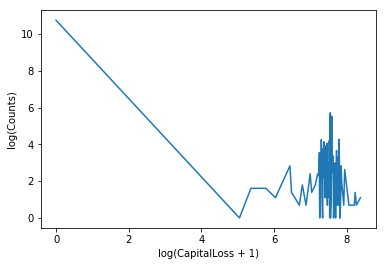Oops, wrong type of plot. Let's try fitting a line and plot the points in a scatter plot.

In :
coeffs = np.polyfit(m[:, 0], m[:, 1], 1)
plt.scatter(m[:, 0], m[:, 1], marker="o")
x = np.arange(0, 10)
plt.plot(x, coeffs * x + coeffs, color="green")
plt.xlabel("log(CapitalLoss + 1)")
plt.ylabel("log(Counts)")

Out:
<matplotlib.text.Text at 0x7fdad3294cf8>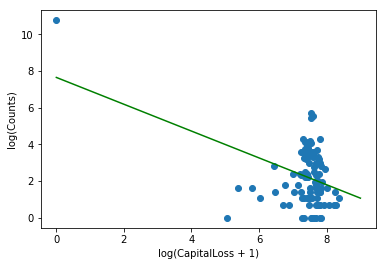Ok, this is pretty much the same too and totally not what we envisioned things to be. Ack.

I recalled seeing at a talk someone plotting a distribution of a single variable grouped by classes, with each curve for a class colored differently. A little googling yields these results: http://stackoverflow.com/a/31258153/732396 http://stackoverflow.com/a/32748510/732396

which we make use of in the plots below:

In :
df.groupby("Income").CapitalLoss.hist(normed=1, alpha=0.6,)

Out:
Income
<=50K    Axes(0.125,0.125;0.775x0.755)
>50K     Axes(0.125,0.125;0.775x0.755)
Name: CapitalLoss, dtype: object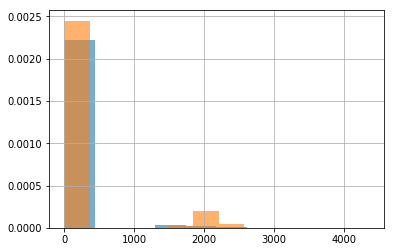In :
df.groupby("Income").CapitalGain.hist(normed=1, alpha=0.6)

Out:
Income
<=50K    Axes(0.125,0.125;0.775x0.755)
>50K     Axes(0.125,0.125;0.775x0.755)
Name: CapitalGain, dtype: object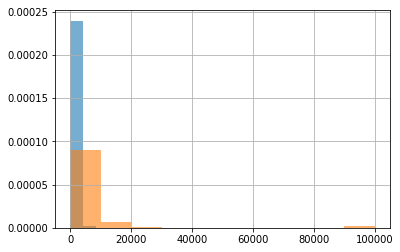I also recall hearing that if there is a marked difference between the distribution of the points between the two classes, then that variable should be a good feature. In this case, what we see from these plots is not exactly great, because for both classes, the majority of the values are concentrated near 0.

Just realized the income isn't at a log scale. Let's take logs.

In :
df2 = df.copy()
df2.CapitalLoss = df2.CapitalLoss + 1
df2.CapitalLoss = df2.CapitalLoss.apply(lambda r: np.log(r + 1))
df2.groupby("Income").CapitalLoss.hist(normed=1, alpha=0.6,)

Out:
Income
<=50K    Axes(0.125,0.125;0.775x0.755)
>50K     Axes(0.125,0.125;0.775x0.755)
Name: CapitalLoss, dtype: object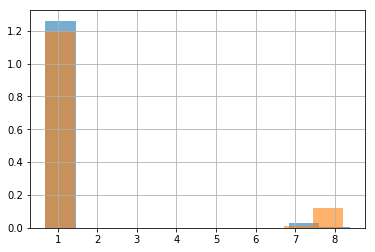In :
df2.CapitalGain = df2.CapitalGain + 1
df2.CapitalGain = df2.CapitalGain.apply(lambda r: np.log(r + 1))
df2.groupby("Income").CapitalGain.hist(normed=1, alpha=0.6,)

Out:
Income
<=50K    Axes(0.125,0.125;0.775x0.755)
>50K     Axes(0.125,0.125;0.775x0.755)
Name: CapitalGain, dtype: object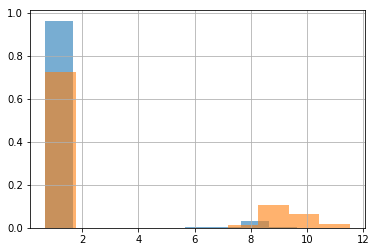The situation is similar. Never mind.

Onto the machine learning. For the CapitalLoss and CapitalGain columns, we will add 1 to all the values and then take logs. This will not leak data from the test set into the training set. Ultimately, we will be using StandardScaler to transform those 2 columns before passing the data to Logistic Regression.

In :
df.CapitalGain = df.CapitalGain + 1
df.CapitalGain = df.CapitalGain.apply(lambda r: np.log(r + 1))
df.CapitalLoss = df.CapitalLoss + 1
df.CapitalLoss = df.CapitalLoss.apply(lambda r: np.log(r + 1))

# Apply what's necessary amongst the stuff we did earlier
df["Income"] = df["Income"].map({ "<=50K": -1, ">50K": 1 })
y_all = df.Income.values
df = pd.get_dummies(df, columns=[
"WorkClass", "Education", "MaritalStatus", "Occupation", "Relationship",
"Race", "Gender", "NativeCountry",
])
# We have to redo the train-test split because of the new columns
df.drop("Income", axis=1, inplace=True,)
X_train, X_test, y_train, y_test = train_test_split(
df, y_all, test_size=0.25, stratify=y_all, random_state=rs,
)

# Note the newly added CapitalGain and CapitalLoss columns
standard_scaler_cols = [
"Age", "fnlwgt", "EducationNum", "CapitalGain", "CapitalLoss", "HoursPerWeek",
]
other_cols = list(set(df.columns) - set(standard_scaler_cols))

# We need to create a new DataFrameMapper and Pipeline object to
# incorporate those 2 columns
mapper = DataFrameMapper(
[([col,], StandardScaler(),) for col in standard_scaler_cols] +
[(col, None,) for col in other_cols]
)
clf = LogisticRegression(random_state=rs,)
pipeline = Pipeline([
("scale", mapper,),
("logit", clf,)
])
strat_kfold = StratifiedKFold(10, random_state=rs,)
estimator = GridSearchCV(
pipeline,
param_grid={
"logit__C": np.power(10, np.arange(-4.0, 5.0)),
"logit__class_weight": ["balanced", None,],
},
scoring=make_scorer(roc_auc_score),
cv=strat_kfold,
)
estimator.fit(X_train, y_train)

Out:
GridSearchCV(cv=StratifiedKFold(n_splits=10,
random_state=<mtrand.RandomState object at 0x7fda6e21e438>,
shuffle=False),
error_score='raise',
estimator=Pipeline(steps=[('scale', DataFrameMapper(default=False, df_out=False,
features=[(['Age'], StandardScaler(copy=True, with_mean=True, with_std=True)), (['fnlwgt'], StandardScaler(copy=True, with_mean=True, with_std=True)), (['EducationNum'], StandardScaler(copy=True, with_mean=True, with_std=True)), (... object at 0x7fda6e21e438>,
solver='liblinear', tol=0.0001, verbose=0, warm_start=False))]),
fit_params={}, iid=True, n_jobs=1,
param_grid={'logit__C': array([  1.00000e-04,   1.00000e-03,   1.00000e-02,   1.00000e-01,
1.00000e+00,   1.00000e+01,   1.00000e+02,   1.00000e+03,
1.00000e+04]), 'logit__class_weight': ['balanced', None]},
pre_dispatch='2*n_jobs', refit=True, return_train_score=True,
scoring=make_scorer(roc_auc_score), verbose=0)
In :
cv_results_df = pd.DataFrame(estimator.cv_results_)

Out:
mean_fit_time mean_score_time mean_test_score mean_train_score param_logit__C param_logit__class_weight params rank_test_score split0_test_score split0_train_score ... split7_test_score split7_train_score split8_test_score split8_train_score split9_test_score split9_train_score std_fit_time std_score_time std_test_score std_train_score
8 0.279067 0.010767 0.817050 0.818658 1 balanced {'logit__C': 1.0, 'logit__class_weight': 'bala... 1 0.815697 0.819107 ... 0.827235 0.816955 0.824332 0.817792 0.812133 0.818966 0.005344 0.000906 0.006772 0.001005
6 0.202885 0.010831 0.816884 0.818323 0.1 balanced {'logit__C': 0.1, 'logit__class_weight': 'bala... 2 0.815307 0.819285 ... 0.825979 0.817276 0.824364 0.817009 0.810812 0.818844 0.003923 0.000269 0.006716 0.001009
10 0.394470 0.010514 0.816636 0.818783 10 balanced {'logit__C': 10.0, 'logit__class_weight': 'bal... 3 0.814980 0.819033 ... 0.826844 0.817105 0.824723 0.817899 0.810992 0.819069 0.031814 0.001020 0.006850 0.001017
14 0.600169 0.010419 0.816540 0.818817 1000 balanced {'logit__C': 1000.0, 'logit__class_weight': 'b... 4 0.814980 0.818973 ... 0.826844 0.817315 0.824723 0.817962 0.811351 0.819089 0.067916 0.000588 0.006799 0.000943
12 0.555784 0.010368 0.816540 0.818803 100 balanced {'logit__C': 100.0, 'logit__class_weight': 'ba... 4 0.814980 0.818973 ... 0.826844 0.817315 0.824723 0.817855 0.811351 0.819089 0.038998 0.000374 0.006799 0.000959

5 rows × 32 columns

In :
print(estimator.best_params_)

{'logit__C': 1.0, 'logit__class_weight': 'balanced'}


As opposed to the case without the CapitalGain and CapitalLoss columns, the regularization parameter is 1.0 here, as opposed to 0.01 .

In :
y_train_predicted = estimator.predict(X_train)
print("Training set accuracy score: {}".format(
accuracy_score(y_train, y_train_predicted)
))
print("Training set AUROC score: {}".format(estimator.score(X_train, y_train)))
print("\nConfusion matrix for training set:")
training_confusion_matrix = confusion_matrix(
y_train, y_train_predicted
)
display(_build_df_from_confusion_matrix(training_confusion_matrix))
print("Same as above but in fractions:")
display(_build_df_from_confusion_matrix(training_confusion_matrix, as_fractions=True))
print("Precision, recall, f-score:")
precision_recall_fscore_support(y_train, y_train_predicted)

Training set accuracy score: 0.8053561191340668
Training set AUROC score: 0.8188093217197174

Confusion matrix for training set:

Predicted <= 50K > 50K
Actual
<= 50K 22098 5768
> 50K 1362 7403
Same as above but in fractions:

Predicted <= 50K > 50K
Actual
<= 50K 0.793009 0.206991
> 50K 0.155391 0.844609
Precision, recall, f-score:

Out:
(array([ 0.94194373,  0.56206818]),
array([ 0.7930094 ,  0.84460924]),
array([ 0.86108405,  0.67496353]),
array([27866,  8765]))

Results from without the CapitalGain and CapitalLoss columns:

Training set accuracy score: 0.785755234637329
Training set AUROC score: 0.8071775040759195

Precision, recall, f-score:

(array([ 0.94135285,  0.53286032]),
array([ 0.76609488,  0.84826013]),
array([ 0.84472934,  0.65454706]),
array([27866,  8765]))



There is an improvement in the precision for the positive class and recall for the positive class, but a drop in recall for the negative class.

In :
y_test_predicted = estimator.predict(X_test)
print("Test set accuracy score: {}".format(
accuracy_score(y_test, y_test_predicted)
))
print("Test set AUROC score: {}".format(estimator.score(X_test, y_test)))
print("\nConfusion matrix for test set:")
test_confusion_matrix = confusion_matrix(y_test, y_test_predicted)
display(_build_df_from_confusion_matrix(test_confusion_matrix))
print("Same as above but in fractions:")
display(_build_df_from_confusion_matrix(test_confusion_matrix, as_fractions=True))
print("Precision, recall, f-score:")
precision_recall_fscore_support(y_test, y_test_predicted)

Test set accuracy score: 0.8048480877896979
Test set AUROC score: 0.818598282440006

Confusion matrix for test set:

Predicted <= 50K > 50K
Actual
<= 50K 7359 1930
> 50K 453 2469
Same as above but in fractions:

Predicted <= 50K > 50K
Actual
<= 50K 0.792227 0.207773
> 50K 0.155031 0.844969
Precision, recall, f-score:

Out:
(array([ 0.94201229,  0.56126392]),
array([ 0.79222737,  0.8449692 ]),
array([ 0.86065142,  0.67449802]),
array([9289, 2922]))

Results from without the CapitalGain and CapitalLoss columns:

Test set accuracy score: 0.7903529604455
Test set AUROC score: 0.8116512329133934

Precision, recall, f-score:

(array([ 0.94322224,  0.53917749]),
array([ 0.77080418,  0.85249829]),
array([ 0.84834123,  0.66056749]),
array([9289, 2922]))



Incorporating the CapitalGain and CapitalLoss columns have improved the accuracy and AUROC scores slightly. Similarly for precision for the positive class. Recall for the positive class dropped slightly but recall for the negative class increased.

Comparing the confusion matrices, we see that by incorporating these 2 columns, there is a decrease in the number of false positives but an increase in the number of false negatives.

Is this a better classifier? Arguably.

## Cheating¶

I definitely need more experience playing around with data sets and machine learning algorithms. Since I already have some stuff here, why not go further? What I want to do:

1. Polynomial Features
2. Feature Selection using PCA
3. Train Logistic Regression on the PCA learned features

NOTE: The stuff in this section can take really long to run on a normal PC and can take up to 16GB of RAM on same occasions. Eventually I decided to spin up a c4.8xlarge EC2 instance on AWS to run this. It costs over \$2 USD an hour just to run the instance and the cross validation took over an hour.

In :
from sklearn.preprocessing import PolynomialFeatures

poly = PolynomialFeatures(degree=2, include_bias=False,)
df_cheat = poly.fit_transform(df)
print(df_cheat.shape)

(48842, 5994)


I wanted to do degree 3 polynomial features but that the memory require exceeded that on my system because dense matrices are required. Using degree 2 polynomial feature transformation, we got a total of 108 (original features) + 108 (original features to 2nd power) + (108 choose 2 = 5778 interaction features) = 5994 features. Wow.\

Let's restore the column names. Code modified from http://stackoverflow.com/a/36735970

In :
df_cheat = pd.DataFrame(data=df_cheat)
df_cheat.columns = [
" * ".join([
("{}^{}".format(col, power) if power > 1 else col)
for col, power in cols_zipped_with_powers if power > 0
])
for cols_zipped_with_powers in [zip(df.columns, p) for p in poly.powers_]
]

Out:
0 25.0 226802.0 7.0 0.693147 0.693147 40.0 0.0 0.0 0.0 0.0 ... 0.0 0.0 0.0 0.0 1.0 0.0 0.0 0.0 0.0 0.0
1 38.0 89814.0 9.0 0.693147 0.693147 50.0 0.0 0.0 0.0 0.0 ... 0.0 0.0 0.0 0.0 1.0 0.0 0.0 0.0 0.0 0.0
2 28.0 336951.0 12.0 0.693147 0.693147 40.0 0.0 0.0 1.0 0.0 ... 0.0 0.0 0.0 0.0 1.0 0.0 0.0 0.0 0.0 0.0
3 44.0 160323.0 10.0 8.947676 0.693147 40.0 0.0 0.0 0.0 0.0 ... 0.0 0.0 0.0 0.0 1.0 0.0 0.0 0.0 0.0 0.0
4 18.0 103497.0 10.0 0.693147 0.693147 30.0 1.0 0.0 0.0 0.0 ... 0.0 0.0 0.0 0.0 1.0 0.0 0.0 0.0 0.0 0.0

5 rows × 5994 columns

Looks correct to me.

We'll do the train-test split before dimensionality reduction using PCA. We'll also do scaling for the columns containing learned features so PCA is not affected by the range of values for the polynomial features.

In :
X_train, X_test, y_train, y_test = train_test_split(
df_cheat, y_all, test_size=0.25, stratify=y_all, random_state=rs,
)
standard_scaler_cols = [
"Age", "fnlwgt", "EducationNum", "CapitalGain", "CapitalLoss", "HoursPerWeek",
] + list(df_cheat.columns[108:])
other_cols = list(set(df_cheat.columns) - set(standard_scaler_cols))

mapper = DataFrameMapper(
[([col,], StandardScaler(),) for col in standard_scaler_cols] +
[(col, None,) for col in other_cols]
)

In :
from sklearn.decomposition import PCA
pca = PCA()
pca.fit(mapper.fit_transform(X_train))

Out:
PCA(copy=True, iterated_power='auto', n_components=None, random_state=None,
svd_solver='auto', tol=0.0, whiten=False)
In :
np.cumsum(pca.explained_variance_ratio_)

Out:
array([ 0.01134274,  0.01871352,  0.02537224, ...,  1.        ,
1.        ,  1.        ])

Let's find the number of components such that the explained variance exceeds 0.9

In :
np.argmax(np.cumsum(pca.explained_variance_ratio_) > 0.9)

Out:
1465

So the first 1466 components explain 90% of the variance. Let's keep that many components.

Because PCA takes such a long time to run and we have to pick the number of components and there is quite a bit of effort involved in writing a custom estimator to do that, I do not want to include PCA in a pipeline for the cross validation step. So we'll just run it. The drawback is that during cross validation, there is some leakage from the training set to the test set. But we'll live with that.

In :
pca2 = PCA(n_components=1466)
X_train_pca = pca2.fit_transform(X_train)

In :
clf = LogisticRegression(random_state=rs,)
strat_kfold = StratifiedKFold(10, random_state=rs,)
estimator = GridSearchCV(
clf,
param_grid={
"C": np.power(10, np.arange(-4.0, 5.0)),
"class_weight": ["balanced", None,],
},
scoring=make_scorer(roc_auc_score),
cv=strat_kfold,
)
estimator.fit(X_train_pca, y_train)

Out:
GridSearchCV(cv=StratifiedKFold(n_splits=10,
random_state=<mtrand.RandomState object at 0x7fda6e21e438>,
shuffle=False),
error_score='raise',
estimator=LogisticRegression(C=1.0, class_weight=None, dual=False, fit_intercept=True,
intercept_scaling=1, max_iter=100, multi_class='ovr', n_jobs=1,
penalty='l2',
random_state=<mtrand.RandomState object at 0x7fda6e21e438>,
solver='liblinear', tol=0.0001, verbose=0, warm_start=False),
fit_params={}, iid=True, n_jobs=1,
param_grid={'C': array([  1.00000e-04,   1.00000e-03,   1.00000e-02,   1.00000e-01,
1.00000e+00,   1.00000e+01,   1.00000e+02,   1.00000e+03,
1.00000e+04]), 'class_weight': ['balanced', None]},
pre_dispatch='2*n_jobs', refit=True, return_train_score=True,
scoring=make_scorer(roc_auc_score), verbose=0)
In :
estimator.best_params_

Out:
{'C': 0.0001, 'class_weight': 'balanced'}
In :
y_train_predicted = estimator.predict(X_train_pca)
print("Training set accuracy score: {}".format(
accuracy_score(y_train, y_train_predicted)
))
print("Training set AUROC score: {}".format(estimator.score(X_train_pca, y_train)))
print("\nConfusion matrix for training set:")
train_confusion_matrix = confusion_matrix(y_train, y_train_predicted)
display(_build_df_from_confusion_matrix(train_confusion_matrix))
print("Same as above but in fractions:")
display(_build_df_from_confusion_matrix(train_confusion_matrix, as_fractions=True))
print("Precision, recall, f-score:")
precision_recall_fscore_support(y_train, y_train_predicted)

Training set accuracy score: 0.7082252736753023
Training set AUROC score: 0.7904336432169127

Confusion matrix for training set:

Predicted <= 50K > 50K
Actual
<= 50K 17633 10233
> 50K 455 8310
Same as above but in fractions:

Predicted <= 50K > 50K
Actual
<= 50K 0.632778 0.367222
> 50K 0.051911 0.948089
Precision, recall, f-score:

Out:
(array([ 0.9748452 ,  0.44814755]),
array([ 0.6327783 ,  0.94808899]),
array([ 0.76741959,  0.60861286]),
array([27866,  8765]))

Numbers for training set without polynomial features and PCA:

Training set accuracy score: 0.8053561191340668
Training set AUROC score: 0.8188093217197174

(array([ 0.94194373,  0.56206818]),
array([ 0.7930094 ,  0.84460924]),
array([ 0.86108405,  0.67496353]),
array([27866,  8765]))



Accuracy dropped by 0.1 and AUROC score dropped slightly by about 0.03 . For the negative class, precision increased but recall and f-score dropped. For the positive class, there is an increase in recall but decrease in precision and f-score.

Now for the test set.

In :
X_test_pca = pca2.transform(X_test)
y_test_predicted = estimator.predict(X_test_pca)
print("Test set accuracy score: {}".format(
accuracy_score(y_test, y_test_predicted)
))
print("Test set AUROC score: {}".format(estimator.score(X_test_pca, y_test)))
print("\nConfusion matrix for test set:")
test_confusion_matrix = confusion_matrix(y_test, y_test_predicted)
display(_build_df_from_confusion_matrix(test_confusion_matrix))
print("Same as above but in fractions:")
display(_build_df_from_confusion_matrix(test_confusion_matrix, as_fractions=True))
print("Precision, recall, f-score:")
precision_recall_fscore_support(y_test, y_test_predicted)

Test set accuracy score: 0.702645156006879
Test set AUROC score: 0.7843801397795291

Confusion matrix for test set:

Predicted <= 50K > 50K
Actual
<= 50K 5830 3459
> 50K 172 2750
Same as above but in fractions:

Predicted <= 50K > 50K
Actual
<= 50K 0.627624 0.372376
> 50K 0.058864 0.941136
Precision, recall, f-score:

Out:
(array([ 0.97134289,  0.44290546]),
array([ 0.62762407,  0.94113621]),
array([ 0.76254006,  0.60234366]),
array([9289, 2922]))

Numbers from the test set without polynomial features + PCA:

Test set accuracy score: 0.8048480877896979
Test set AUROC score: 0.818598282440006
(array([ 0.94201229,  0.56126392]),
array([ 0.79222737,  0.8449692 ]),
array([ 0.86065142,  0.67449802]),
array([9289, 2922]))



This is the same situation as for the training set. So overall, polynomial features followed by PCA actually did worse in terms of precision and f-score for the positive class, but performed better in terms of recall.

In [ ]:



Disclaimer: Opinions expressed on this blog are solely my own and do not express the views or opinions of my employer(s), past or present.